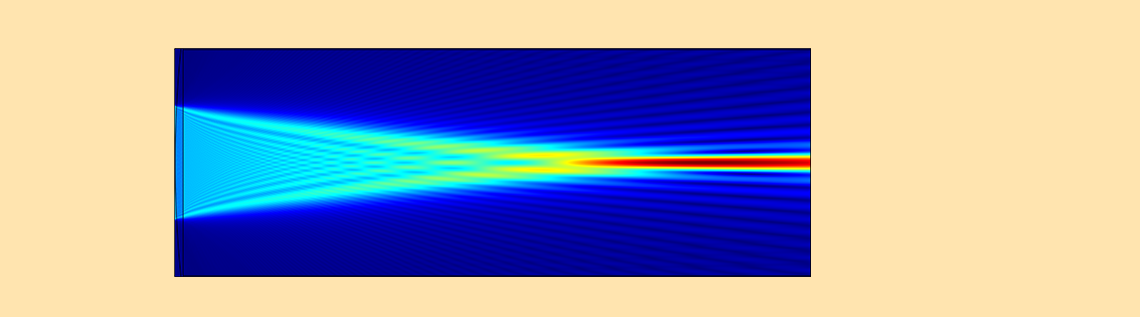# How to Perform Lens Simulations Using the Wave Optics Module

February 19, 2020

Performing lens simulations in wave optics is generally difficult, because it requires a lot of mesh elements. In this blog post, we demonstrate how the Wave Optics Module, an add-on to the COMSOL Multiphysics® software, can be used to perform lens simulations based on Maxwell’s equations.

### Introduction to the Optics Simulation Methods

There are two main categories of methods to simulate optics:

1. Ray tracing
2. Wave optics

If you want to simulate the diffraction effect (even simply focusing a beam introduces diffraction), wave optics is needed. In wave optics, we consider two types of methods: a full-wave Maxwell method and a beam propagation method (BPM). Each method has certain limitations, outlined below:

Method Theoretical Exactness Diffraction Reflection Computational Effort
Ray tracing Approximate No Yes Low
Wave optics: full-wave Maxwell (conventional) Rigorous Yes Yes High
Wave optics: BPM (Fraunhofer, Fresnel, others) Approximate Yes No Medium

Ray tracing is an approximation where the wavelength is negligible compared to the object size; therefore, it doesn’t deal with diffraction. The full-wave Maxwell solver literally solves Maxwell’s equations, so it’s rigorous and there is theoretically no model approximation. The BPM typically includes various approximations in the formulation, such as the Fraunhofer approximation (i.e., Fourier transformation) and the Fresnel diffraction formula.

The full-wave Maxwell solver looks like the greatest method. However, when it comes to optics simulation, there is a problem with the “conventional” Maxwell solver: It requires a fine mesh and lot of memory to solve:

• Optical components
• Interference patterns due to surface reflections

In the conventional Maxwell solver, all points within the computational domain contribute when running the simulation. For this reason, we need a mesh throughout the domain, and the mesh elements need to resolve the wavelength. Then, it becomes problematic when you want to simulate a large object, like standard optical lenses. The BPM doesn’t have this problem, because the field solution literally propagates (or jumps) from one plane to another by using a certain propagation law, in which you don’t need a mesh between the planes.

### Lens Simulations with the Beam Envelopes Interface

Compared to conventional Maxwell solvers, the Beam Envelopes interface in the COMSOL® software doesn’t have this difficulty, because the fast oscillation part is factored out in the formulation. You can use this interface if the solution’s envelopes are slowly varying. In practice, there are many such cases. Using this method, you don’t need a lot of mesh elements. So, if we always simulate homogeneous domains and solve for a solution that has slowly varying envelopes, then we are done with this interface. But that’s not all…

We frequently want to solve optical systems that include inhomogeneous domains. The problem we face is the reflection from a material interface; a lens surface, for example. In which way do reflections create a problem? Reflections are also solutions to Maxwell’s equations. So, the Maxwell solver tries to find a solution including reflections if there are some material interfaces. If any reflections occur, they may constructively interfere with the incident beam ending up with standing waves with a half of the wavelength. This worsens the original problem. We need to resolve the half wavelength, which means many more mesh elements! This reduces the value of the Beam Envelopes interface. This situation is shown in the figure below.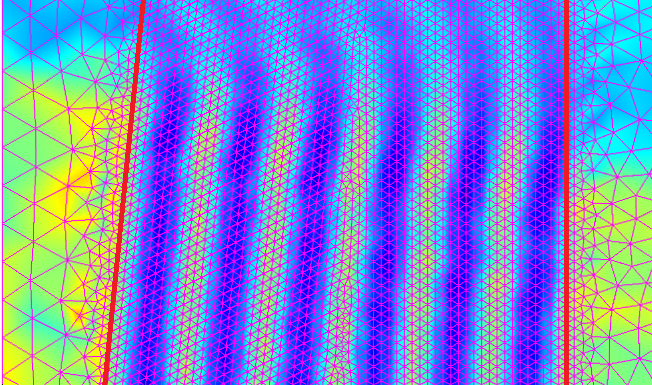A closeup of a lens simulation with the Beam Envelopes interface. There is interference in the lens (center part), which requires finer mesh.

To avoid the surface reflection, we can consider an antireflection (AR) coating, just as each optic has an AR coating in most cases in practice. Let’s take a look at an example of a Gaussian beam that propagates in a free air space, using the Gaussian Beam feature with the Matched boundary condition.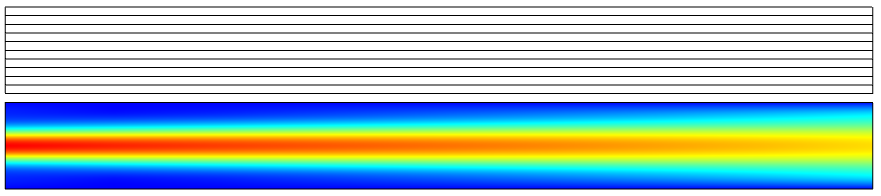Top: The mesh used in the simulation. Only one mesh element is needed along the propagation direction (from left to right). Bottom: The Gaussian beam simulation with the Beam Envelopes interface, the unidirectional formulation. No material other than the air is inserted.

In the above simulation, we put the focus on the left boundary so the beam expands toward the right boundary. All other boundaries besides the left boundary are set to the Matched boundary condition with no excitation. Next, let’s add a glass on the right side of the domain. We expect a reflection in the left side of the domain and no reflection in the right side, so we have to increase the mesh in the left side, but we can keep the single mesh in the right side. (Note that you can still capture the reflection accurately with only one mesh element by using the bidirectional formulation. We are not interested in reflection here, so we stick with the unidirectional formulation.)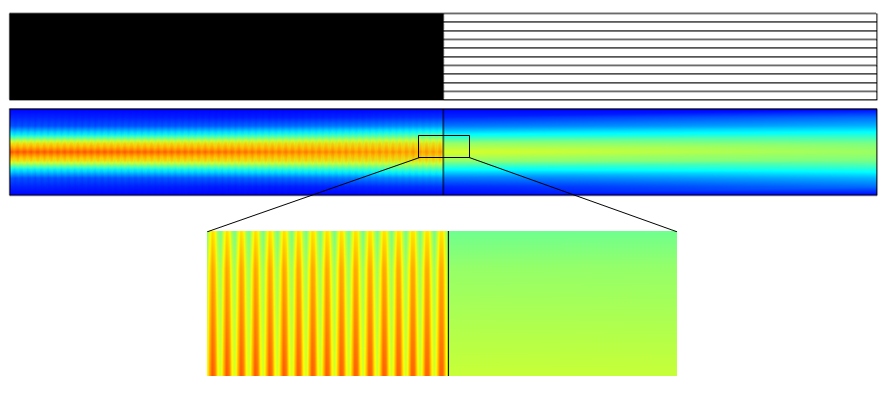The Gaussian beam simulation with the Beam Envelopes interface, the unidirectional formulation. A glass is inserted in the right part of the domain. Shown above is the mesh. Many mesh elements are needed to resolve the half wavelength of the interference pattern, whereas only one mesh is needed in the right side of the domain.

As expected, we get a reflection in the left side of the domain, so we need a lot of mesh elements there. This hurts the advantage of the Beam Envelopes interface. Now, let’s add an AR coating on the glass surface. The simplest AR coating for a monochromatic light is the quarter-lambda AR coating (refer to Optics by Hecht). The quarter-lambda AR coating is a thin film with the refractive index of \sqrt{n_1 n_2}, where n_1 and n_2 are the index for each material sandwiching the film and with the thickness of \lambda_0/\sqrt{n_1 n_2}/4, where \lambda_0 is the vacuum wavelength. With this film, the reflectance becomes zero at \lambda_0. Let’s now see how we can include the AR coating in the simulation.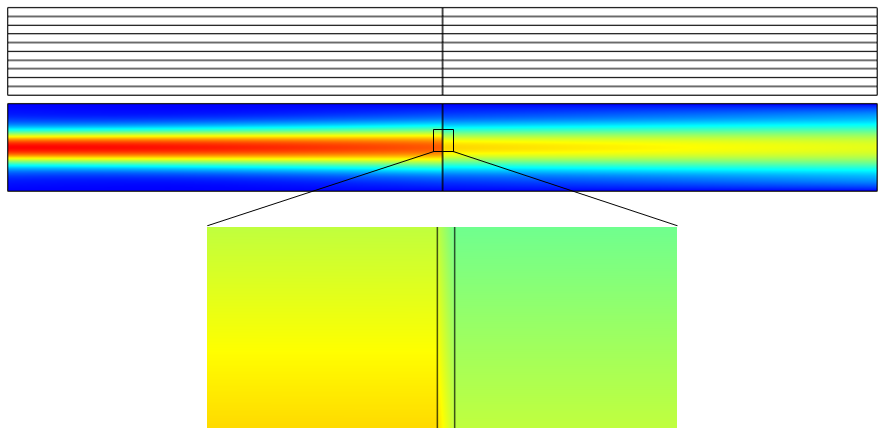The Gaussian beam simulation with the Beam Envelopes interface, the unidirectional formulation. A glass is inserted in the right part of the domain. A quarter-lambda AR coating is put on the glass surface. Shown above is the mesh. Only one mesh is needed for all material domains.

Thanks to the AR coating, there is no longer any reflection, which gives the maximum benefit from this interface. We only need one mesh element in the propagating direction for each domain representing air, AR coating, and glass, respectively.

A boundary condition, introduced as of COMSOL Multiphysics version 5.4, the Transition boundary condition, mimics this quarter-lambda AR coating with zero thickness. All you need to do is to specify the refractive index and the thickness of the coating, which are \sqrt{n_1 n_2} and \lambda_0/\sqrt{n_1 n_2}/4, as in the below figure.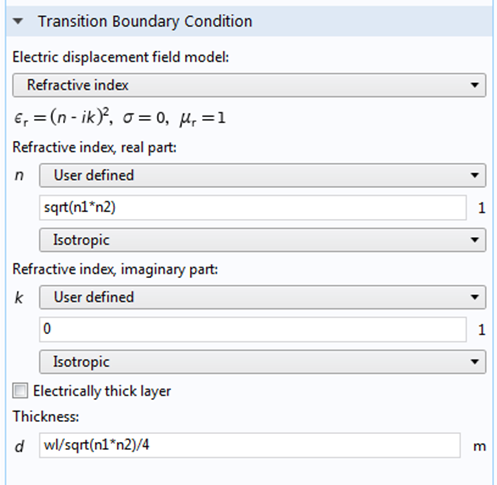Settings of the Transition boundary condition.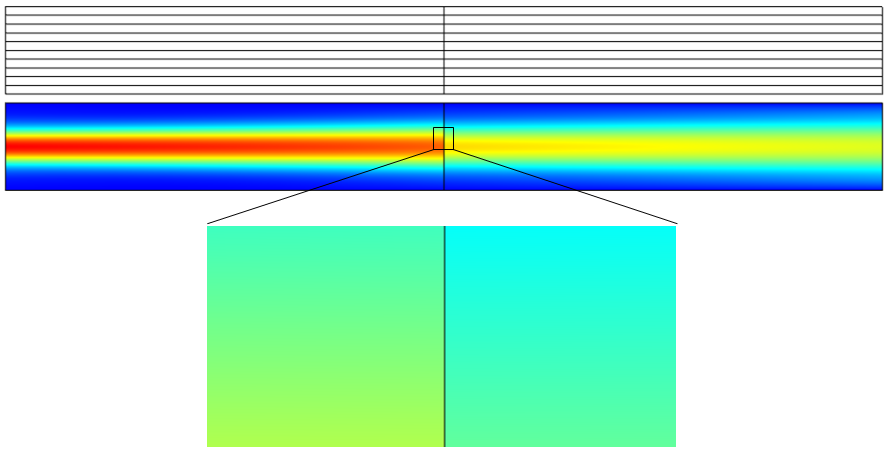The Gaussian beam simulation with the Beam Envelopes interface, the unidirectional formulation. A glass is inserted in the right part of the domain. The Transition boundary condition is applied to the glass surface. Shown above is the mesh. Only one mesh is needed for all domains, since there are no reflections.

Now you can see how the Transition boundary condition is useful, particularly when there are many material interfaces, where you can avoid making a real thin-film coating on each surface.

Finally, with this boundary condition, the first figure of the lens simulation with a lot of interference becomes completely clean, as shown below.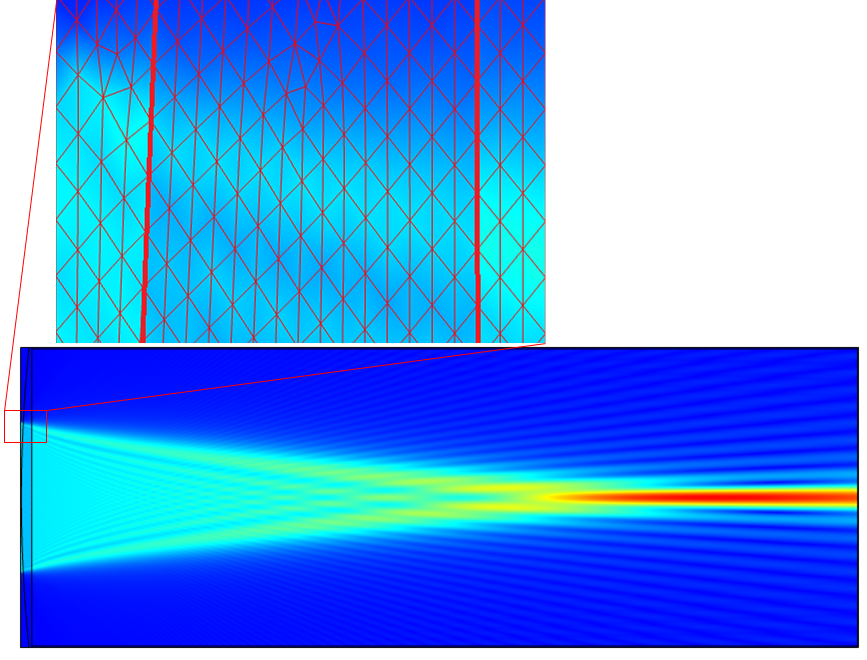A lens simulation with the Beam Envelopes interface, the unidirectional formulation, and the Transition boundary condition. There is no reflection causing interference, which enables a coarser mesh.

### Concluding Remarks

Conventional full-wave Maxwell solvers require a lot of mesh elements when used for optics simulation. The Beam Envelopes interface, the unidirectional formulation, and the Transition boundary condition together address this problem under certain conditions. We can use this approach for large optical systems that include optics and even for multioptical systems couplings. Such applications include lens systems, waveguides, external optical systems, fiber couplings, laser diode stacks, and laser beam delivery systems.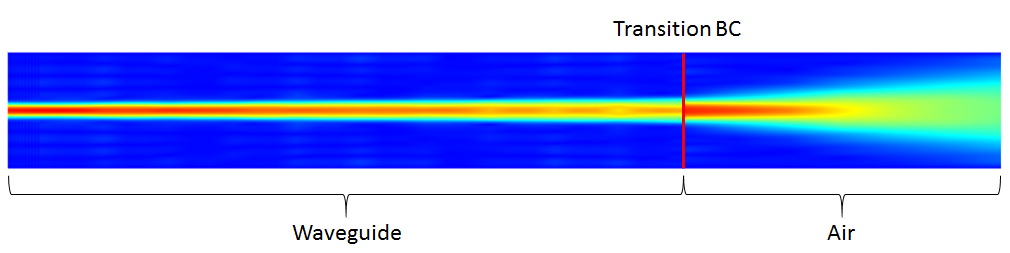Simulation of a waveguide that out-couples to the ambient air.

### Next Step

See how the Wave Optics Module can benefit your lens simulations by clicking the button below:

#### Categories##### Xi Shen
May 2, 2022##### Yosuke Mizuyama
May 3, 2022 COMSOL Employee

Hi Shen,
Thank you for reading this blog.